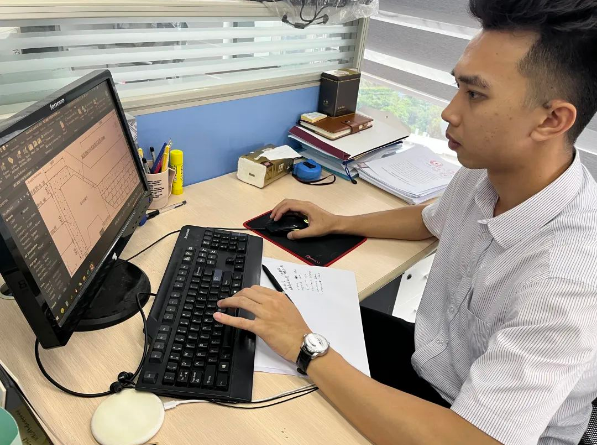# 【致诚风采】2022致诚6月份优秀员工风采专题报道

-----李大钊

<section style="margin: 0px; padding: 0px; outline: 0px; max-width: 100%; font-family: system-ui, -apple-system, BlinkMacSystemFont, " helvetica="" neue",="" "pingfang="" sc",="" "hiragino="" sans="" gb",="" "microsoft="" yahei="" ui",="" yahei",="" arial,="" sans-serif;="" font-size:="" 17px;="" letter-spacing:="" 0.544px;="" background-color:="" rgb(254,="" 254,="" 254);="" text-indent:="" 2em;="" visibility:="" visible;="" box-sizing:="" border-box="" !important;="" overflow-wrap:="" break-word="" !important;"="">